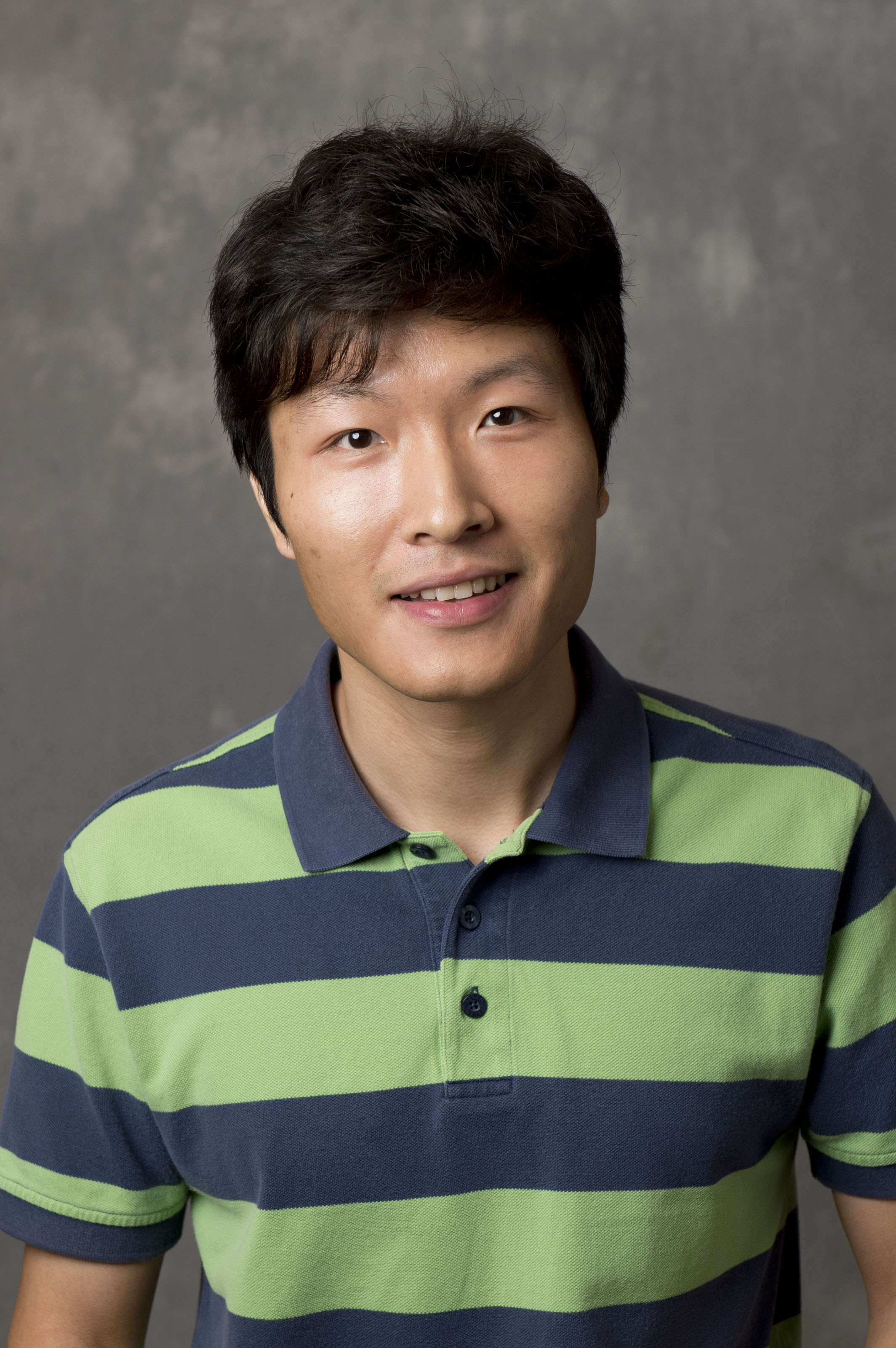# Yiran Wang

Welcome! I am an Assistant Professor at Department of Mathematics, Emory University. Before, I was a Postdoc Scholar at Stanford University working with Prof. András Vasy. I also worked with Prof. Gunther Uhlmann as an Acting Assistant Professor at University of Washington (with a joint position at IAS, HKUST). I obtained my Ph.D from Purdue University under the supervision of Prof. Antônio Sá Barreto.

My research interests are microlocal analysis, inverse problems, integral geometry, spectral and scattering theory.

Here is my contact information:
• E-mail: yiran.wang(at)emory.edu

• Office: Mathematics & Science Center, E414.

Department of Mathematics
400 Dowman Drive
Atlanta, GA 30322### Teaching

In 2022 Fall semester, I will teach In 2022 Spring semester, I am teaching In 2021 Fall semester, I taught Math 545: Introduction to Differential Geometry I.

In 2021 Spring semester, I taught Math 250: Foundations of Mathematics.

In 2020 Fall semester, I taught In 2020 Spring semester, I taught Math 250: Foundations of Mathematics.

In 2019 Fall semester, I taught Math 112z: Calculus II.

### Research

• Recovery of black hole mass from a single quasinormal mode (with G. Uhlmann)
Submitted.

• Cosmic background radiation in scalar gravity
Preprint.

• Existence of resonances for Schrödinger operators on hyperbolic space (with D. Borthwick)
Submitted.

• Inverse problems for the Boltzmann equation in cosmology
Preprint.

• Some integral geometry problems for wave equations
Inverse Problems.
(Special issue of Emerging Talents)

• Microlocal analysis of the light ray transform on globally hyperbolic Lorentzian manifolds
Submitted.

• The anisotropic Calderón problem for high fixed frequency (with G. Uhlmann)
Submitted.

• Inverse scattering for critical semilinear wave equations (with A. Sá Barreto, G. Uhlmann)
Pure and Applied Analysis.

• Streak artifacts from non-convex metal objects in X-ray tomography (with Y. Zou)
Pure and Applied Analysis.

• Sharp resolvent estimates on non-positively curved asymptotically hyperbolic manifolds
International Mathematics Research Notices (IMRN).

• On the light ray transform of wave equation solutions (with A. Vasy)
Communications in Mathematical Physics.

• Convolutional neural networks in phase space and inverse problems (with G. Uhlmann)
SIAM Journal on Applied Mathematics.

• Singularities generated by the triple interaction of semilinear conormal waves (with A. Sá Barreto)
Analysis and PDE.

• Determination of space-time structures from gravitational perturbations (with G. Uhlmann)
Communications on Pure and Applied Mathematics.
(Top cited article 2020-2021)

• Nonlinear interaction of waves in elastodynamics and an inverse problem (with M. de Hoop, G. Uhlmann)
Mathematische Annalen.

• Quantitavie analysis of metal artifacts in X-ray tomography (with B. Palacios, G. Uhlmann)
SIAM Journal on Mathematical Analysis.

• Nonlinear responses from the interaction of two progressing waves at an interface (with M. de Hoop, G. Uhlmann)
Annales de l'Institut Henri Poincaré, Analyse Non Linéaire.

• Determination of vacuum space-times from the Einstein-Maxwell equations (with M. Lassas, G. Uhlmann)
Submitted.

• Distribution of resonances for analytic asymptotically hyperbolic spaces
Journal of Spectral Theory.

• Parametrices for the light ray transform on Minkowski spacetime
Inverse Problems and Imaging.

• Inverse problems for quadratic derivative nonlinear wave equations (with T. Zhou)
Communications in Partial Differential Equations.

• Reducing streaking artifacts in quantitative susceptibility mapping (with B. Palacios, G. Uhlmann)
SIAM Journal of Imaging Sciences.

• The scattering operator on asymptotically hyperbolic manifolds (with A. Sá Barreto)
Journal of Spectral Theory.

• Inverse problems for semilinear wave equations on Lorentzian manifolds (with M. Lassas, G. Uhlmann)
Communications in Mathematical Physics.

• The semiclassical resolvent on conformally compact manifolds with variable curvature at infinity (with A. Sá Barreto)
Communications in Partial Differential Equations.

• Resolvent and radiation fields on non-trapping asymptotically hyperbolic manifolds

• ### Recent and upcoming presentations:

• Workshop "Scattering and Inverse Problems", Linz, Austria, 10-2022.
• Workshop "Geometrical Inverse Problems", Linz, Austria, 11-2022.
• Workshop "Inverse Problems in the Desert", New York University Abu Dhabi, 12-2022.
• BIRS Workshop "Inverse Problems and Nonlinearity", Banff, Canada, 07-2023.

• Last updated: 08-2022.